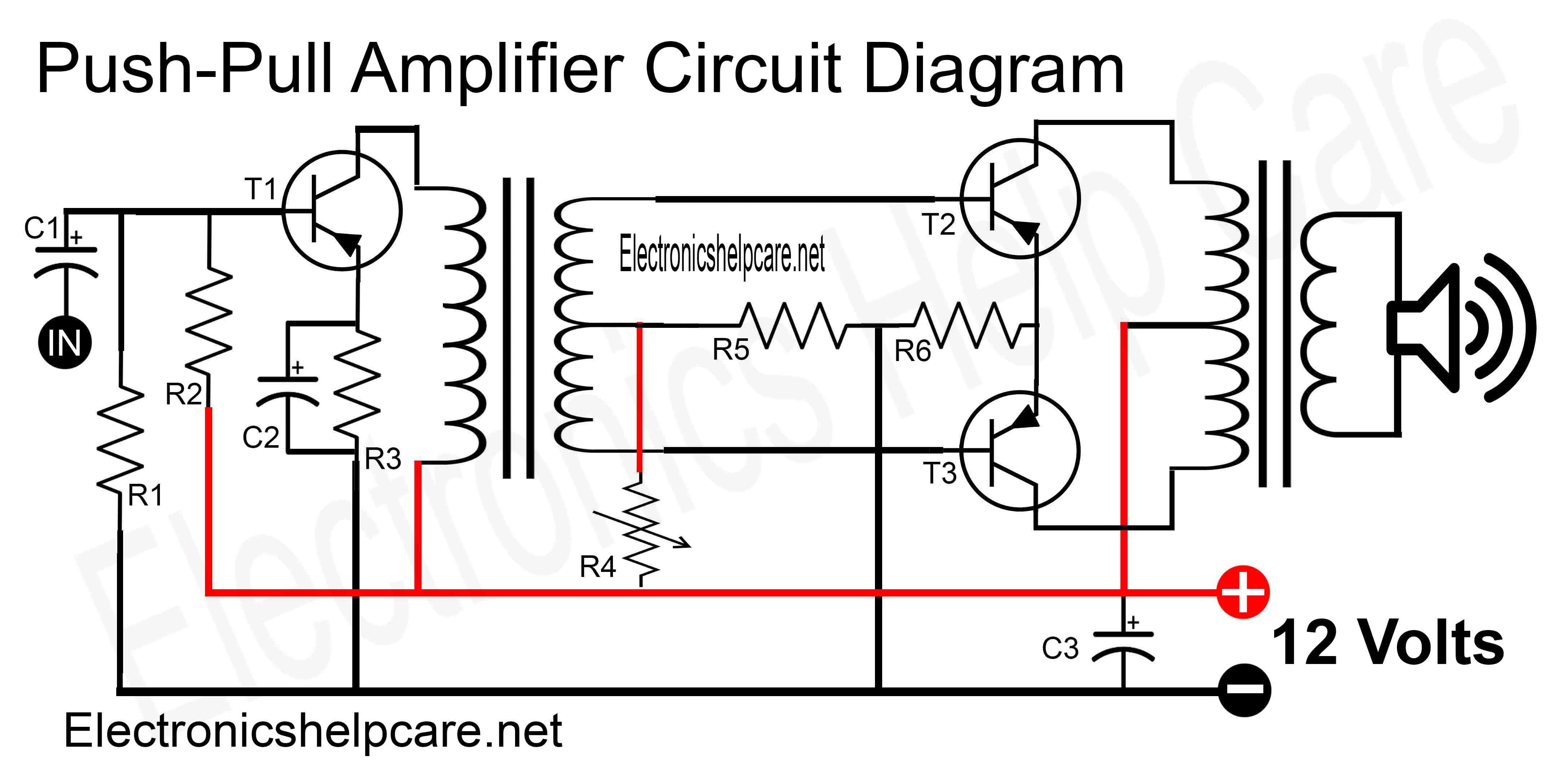Breaking News

# Push-Pull amplifier circuit diagram

## Push-Pull amplifier circuit diagram:

This is a Push-Pull amplifier circuit diagram. it has two transformers. One for the audio input and another transformer for the audio output. here used 3 transistors. all transistors are PNP transistors. it run with 12 voltage. 12 voltage transformer and 12 voltage battery can be used.Component list for this circuit.

R1=500 ohms. R2=6k8. R3=50 ohms. R4=3k. R5=10 ohms. R6=1ohms. C1=10uf/25 volts. C2=100uf/25volts. C3=1000uf/25volts. T1=2sb472. T2=2sb472. T3=2sb472.

Build a Transformer or construct the transformer. Home Theater Circuit Diagram 5.1

Thanks a lot for being with us. If you want another post then please visit our website.

We have another post for you. like repairing

or  Diagram